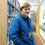# Trig identities of the complex kind

I'm sure most of you have heard of trig identities involving $\sin(x)$, $\cos(x)$,$\tan(x)$, etc...

But what about complex trigonometry? Specifically the trig function $\text{cis}(x)$.

This function is short hand for $\boxed{\cos(x) + i\sin(x)}$ for those of you who haven't met it before. It's used mainly to represent and calculate complex numbers.

So what happens if you add two $\text{cis}(x)$ functions together? What about subtraction, multiplication, division, how does this all affect the $\text{cis}(x)$ function?

Before we get started, I'm going to list the identities I already know which involve this function. These and all future identities will be in boxes.

$\large \boxed{\text{cis}(x) = e^{xi}}$

$\large \boxed{(\text{cis}(x))^n = \text{cis}(nx)}$

Let's first expand on that second one. What if we replace $n$ with a complex number?

$(\text{cis}(x))^{a + bi}$

Knowing that $\boxed{\text{cis}(x) = e^{xi}}$ really helps here.

$(e^{xi})^{a + bi}$

$e^{(a + bi)xi}$

$e^{axi - bx}$

$\frac{e^{axi}}{e^{bx}}$

$\frac{\text{cis}(ax)}{e^{bx}}$

$\large \boxed{(\text{cis}(x))^{a + bi} = \frac{\text{cis}(ax)}{e^{bx}}}$

So now that that's updated we can go on to find new identities.

$\text{cis}(x)\text{cis}(y)$

$e^{xi}e^{yi}$

$e^{(x + y)i}$

$\text{cis}(x + y)$

$\large \boxed{\text{cis}(x)\text{cis}(y) = \text{cis}(x + y)}$

Now division.

$\frac{\text{cis}(x)}{\text{cis}(y)}$

$\frac{e^{xi}}{e^{yi}}$

$e^{xi}e^{-yi}$

$e^{(x - y)i}$

$\text{cis}(x - y)$

$\large \boxed{\frac{\text{cis}(x)}{\text{cis}(y)} = \text{cis}(x - y)}$

Alright so those are done now. Next is addition and subtraction.

$\text{cis}(x) + \text{cis}(y)$

$\text{cis}(x)\left(1 + \frac{\text{cis}(y)}{\text{cis}(x)}\right)$

$\text{cis}(x)(1 + \text{cis}(y - x))$

$\large \boxed{\text{cis}(x) + \text{cis}(y) = \text{cis}(x)(1 + \text{cis}(y - x))}$

And finally subtraction.

$\text{cis}(x) - \text{cis}(y)$

$\text{cis}(x)\left(1 - \frac{\text{cis}(y)}{\text{cis}(x)}\right)$

$\text{cis}(x)(1 - \text{cis}(y - x))$

$\large \boxed{\text{cis}(x) - \text{cis}(y) = \text{cis}(x)(1 - \text{cis}(y - x))}$

That's all for now, if there's any you think I've missed, please tell.

Edit: I forgot one: $\text{arccis}(x)$

$\text{cis}(x) = e^{xi}$

$\ln{\text{cis}(x)} = xi$

$\frac{\ln{\text{cis}(x)}}{i} = x$

$\frac{i\ln{\text{cis}(x)}}{-1} = x$

$-i\ln{\text{cis}(x)} = x$

$x = -i\ln{\text{cis}(x)}$

Replace $x$ with $\text{arccis}(x)$ and $\text{cis}(x)$ with $x$

$\large \boxed{\text{arccis}(x) = -i\ln{x}}$

Here's the new list of identities:

$\large \boxed{\text{cis}(x) = e^{xi}}$

$\large \boxed{(\text{cis}(x))^{a + bi} = \frac{\text{cis}(ax)}{e^{bx}}}$

$\large \boxed{\text{cis}(x)(\text{cis}(y))^{\pm 1} = \text{cis}(x \pm y)}$

$\large \boxed{\text{cis}(x) \pm \text{cis}(y) = \text{cis}(x)(1 \pm \text{cis}(y - x))}$

$\large \boxed{\text{arccis}(x) = -i\ln{x}}$Note by Jack Rawlin
4 years, 5 months ago

This discussion board is a place to discuss our Daily Challenges and the math and science related to those challenges. Explanations are more than just a solution — they should explain the steps and thinking strategies that you used to obtain the solution. Comments should further the discussion of math and science.

When posting on Brilliant:

• Use the emojis to react to an explanation, whether you're congratulating a job well done , or just really confused .
• Ask specific questions about the challenge or the steps in somebody's explanation. Well-posed questions can add a lot to the discussion, but posting "I don't understand!" doesn't help anyone.
• Try to contribute something new to the discussion, whether it is an extension, generalization or other idea related to the challenge.

MarkdownAppears as
*italics* or _italics_ italics
**bold** or __bold__ bold
- bulleted- list
• bulleted
• list
1. numbered2. list
1. numbered
2. list
Note: you must add a full line of space before and after lists for them to show up correctly
paragraph 1paragraph 2

paragraph 1

paragraph 2

[example link](https://brilliant.org)example link
> This is a quote
This is a quote
    # I indented these lines
# 4 spaces, and now they show
# up as a code block.

print "hello world"
# I indented these lines
# 4 spaces, and now they show
# up as a code block.

print "hello world"
MathAppears as
Remember to wrap math in $$ ... $$ or $ ... $ to ensure proper formatting.
2 \times 3 $2 \times 3$
2^{34} $2^{34}$
a_{i-1} $a_{i-1}$
\frac{2}{3} $\frac{2}{3}$
\sqrt{2} $\sqrt{2}$
\sum_{i=1}^3 $\sum_{i=1}^3$
\sin \theta $\sin \theta$
\boxed{123} $\boxed{123}$

Sort by:

This is a great introduction to complex trigonometric functions. Can you add that to the page?

Staff - 4 years, 5 months ago